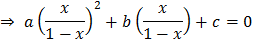# Ifare the roots of, then the equationhas roots a)b)c)d)## Question ID - 103317 :- Ifare the roots of, then the equationhas roots a)b)c)d)3537

(c)

We have,...(i)Also,be the roots of.andHence,andare the required roots.

Next Question :
 What type of colloidal system is fog? (a) Gas is liquid (b) Liquid in gas (c) Liquid in liquid (d) Solid in gas